# Metric transitivity

(diff) ← Older revision | Latest revision (diff) | Newer revision → (diff)

of a dynamical system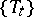with a (quasi-) invariant measureThe property ofthat any measurable subsetof the phase spacewhich is invariant relative to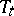(in the sense that it coincides with its complete inverse images) either has measure zero or coincides withup to a set of measure zero. A formally stronger version of this property is obtained if in the definition instead of invariant sets one considers sets which are invariant modulo 0 (a setdiffering for eachfromby a set of measure zero). These two versions are equivalent ifis a-finite measure and if the "time"runs through a locally compact group with a countable base (see the proof for flows in ), but for arbitrary transformation groups this is not the case (see the example, given for another reason, in ).

Instead of metric transitivity one may also speak of metric indecomposability, or ergodicity. If several (quasi-) invariant measures are considered in a given system andis one of them, then instead of speaking of metric transitivity (ergodicity) relative toone speaks of metric transitivity (ergodicity) of. Of course, decomposability of a normalized invariant measuremeans something different, i.e. the possibility to representas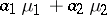, whereare normalized invariant measures different fromand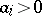. Indecomposability ofis equivalent to the second (stronger) version of metric transitivity (see , ).

If there is a topology on, then under natural assumptions (see the discussion for flows in ) metric transitivity implies that for almost-allthe trajectory ofis everywhere dense (in this sense, metric transitivity implies topological transitivity). The converse is false.

Starting with J. von Neumann , a number of results were obtained concerning the decomposition of non-ergodic systems into ergodic components. For the traditional, purely metric version (a Lebesgue space and only one (quasi-) invariant measure involved) see , , . N.M. Krylov and N.N. Bogolybov  obtained stronger results for topological flows and cascades on a metric compactum, of a twofold kind (see also , ): 1) a decomposition of normalized invariant measures, compatible with the topology, into ergodic measures; and 2) a "geometric" realization of all these decompositions at once by means of ergodic sets (cf. Ergodic set). Regarding the generalization of these results to other transformation groups, it is known that 1) is valid under broader assumptions than 2) (see , ). There are also similar results for dynamical systems (at present only cascades and flows) in appropriate measurable spaces (cf. Measurable space) and (or) for quasi-invariant measures (see ).

Metric transitivity of a partitionof a measure spaceis the property that any measurable subset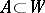consisting entirely of elements ofeither has measure zero or coincides withup to a set of measure zero. Instead of metric transitivity one also speaks of absolute non-measurability of. Metric transitivity of a dynamical system generated by a group of transformations, whose trajectories form a partition of the phase space, coincides with metric transitivity of this partition. If the transformations forming the dynamical system are not invertible, then their metric transitivity also reduces to that of some partition; however, its description is more complicated: the element containing some point consists of all images of the point and all inverse images of these images.

How to Cite This Entry:
Metric transitivity. Encyclopedia of Mathematics. URL: http://encyclopediaofmath.org/index.php?title=Metric_transitivity&oldid=17732
This article was adapted from an original article by D.V. Anosov (originator), which appeared in Encyclopedia of Mathematics - ISBN 1402006098. See original article